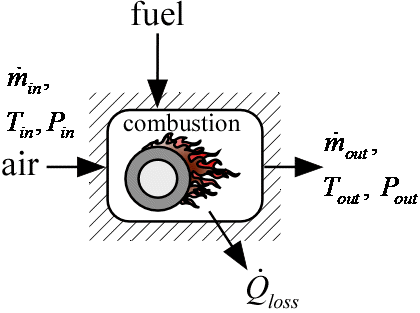Contents - index

Combustor2_CLProcedure Combustor2_CL provides a model of a combustor. It accepts the name of the fuel, which must be a name contained in the list of ideal and NASA gases. The procedure assumes complete combustion with dry air. The percentage of excess air above the stoichiometric requirement is provided in input Excess%. The combustion process is assumed to be adiabatic if eta is 1. If eta is less than 1, then some of the energy provided in the combustion process is assumed to be lost to the surrounding through the combustor jacket.  The loss is calculated using the lower heating value of the fuel as the basis; that is (1-eta)*Q_dot_f is the jacket loss.   The fuel is assumed to be supplied at a reference temperature of 25°C (77°F). The procedure calculates the outlet temperature of the products.  The procedure also returns the mass flow rate of the fluid multiplied by its lower heating value as well as the rate at which energy would be transferred if the products were cooled from their outlet temperature to the inlet temperature of the air used for combustion.

Inputs:

T_in:  inlet temperature of air provided for combustion (K, C, F, or R)

P_in:  inlet pressure of air provided for combustion (bar, atm, Pa, kPa, MPa)

m_dot_in:  inlet mass flow rate of air provided for combustion (kg/s or lb_m/hr)

F\$:  name of the fuel.  Must be in the list of ideal or NASA gases and must only contain C, H, and O.

Excess%:  excess air percent.  Supply 0 [%] for stoichiometric reaction

eta:   fraction of the energy released in the combustion process that appears in the products

DPoverP:  pressure loss in combustor normalized by inlet pressure

Outputs

m_dot_out:  outlet mass flow rate of combustion products (kg/s or lb_m/hr)

P_out:  outlet pressure  of combustion products (bar, atm, Pa, kPa, MPa)

T_out:  outlet temperature of combustion products (K, C, F, or R)

Q_dot_f:  lower heating value of fuel multiplied by fuel mass flow rate (W, kW or Btu/hr)

Q_dot_loss:  rate of heat transfer lost to ambient (W, kW, or Btu/hr)

Q_dot_b:  rate of heat transfer if products were cooled to inlet temperature (W, kW, or Btu/hr)

Example:

\$UnitSystem SI Mass J K Pa

T_in=400 [K] "inlet temperature"

P_in=200000 [Pa] "inlet pressure"

m_dot_in=0.05 [kg/s] "mass flow rate"

F\$='C4H10,n-butane' "fuel name"

Excess%=150 [%] "% excess air"

eta=0.97 "efficiency"

DPoverP=0.02 "nondimensional pressure drop"

Call combustor2_cl(T_in, P_in, m_dot_in, F\$, Excess%, eta, DPoverP: m_dot_out, T_out, P_out, Q_dot_f, Q_dot_loss, Q_dot_b)

AF=m_dot_in/(m_dot_out-m_dot_in) "air-fuel ratio"

{Solution:

AF=38.55

m_dot_out=0.0513 [kg/s]

P_out=196,000 [Pa]

Q_dot_b=57,215 [W]

Q_dot_f=59,297 [W]

Q_dot_loss=1,779 [W]

T_out=1,357 [K]

}